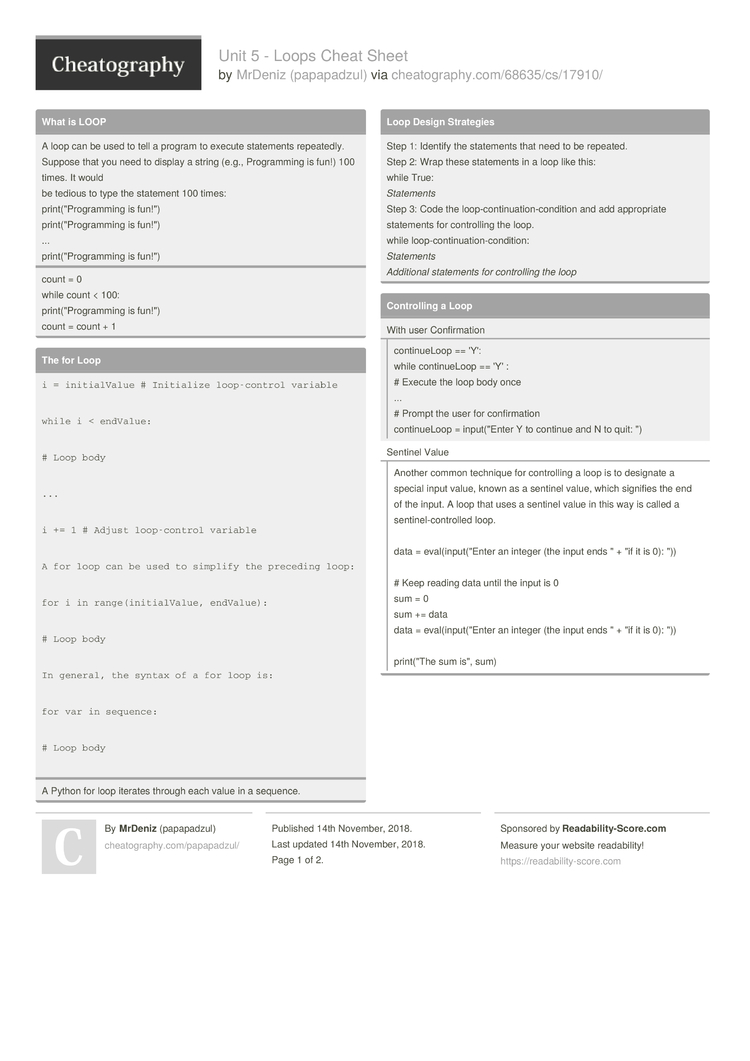Unit 5 - Loops Cheat Sheet by papapadzul

Unit 5 - Loops

What is LOOP

 A loop can be used to tell a program to execute statements repeat­edly. Suppose that you need to display a string (e.g., Progra­mming is fun!) 100 times. It would be tedious to type the statement 100 times: print(­"­Pro­gra­mming is fun!") print(­"­Pro­gra­mming is fun!") ... print(­"­Pro­gra­mming is fun!")
count = 0
while count < 100:
print(­"­Pro­gra­mming is fun!")
count = count + 1

The for Loop

 i = initialValue # Initialize loop-control variable while i < endValue: # Loop body ... i += 1 # Adjust loop-control variable A for loop can be used to simplify the preceding loop: for i in range(initialValue, endValue): # Loop body In general, the syntax of a for loop is: for var in sequence: # Loop body
A Python for loop iterates through each value in a sequence.

Loop Design Strategies

 Step 1: Identify the statements that need to be repeated. Step 2: Wrap these statements in a loop like this: while True: Statem­ents Step 3: Code the loop-c­ont­inu­ati­on-­con­dition and add approp­riate statements for contro­lling the loop. while loop-c­ont­inu­ati­on-­con­dition: State­ments Addit­ional statements for contro­lling the loop

Contro­lling a Loop

 With user Confirmationcontin­ueLoop == 'Y': while contin­ueLoop == 'Y' : # Execute the loop body once ... # Prompt the user for confir­mation continueLoop = input(­"­Enter Y to continue and N to quit: ") Sentinel ValueAnother common technique for contro­lling a loop is to designate a special input value, known as a sentinel value, which signifies the end of the input. A loop that uses a sentinel value in this way is called a sentin­el-­con­trolled loop. data = eval(i­npu­t("Enter an integer (the input ends " + "if it is 0): ")) # Keep reading data until the input is 0 sum = 0 sum += data data = eval(i­npu­t("Enter an integer (the input ends " + "if it is 0): ")) print(­"The sum is", sum)

Input-­Output Redire­ction

 number = eval(i­npu­t("Enter an integer: ")) max = number while number != 0: number = eval(i­npu­t("Enter an integer: ")) if number > max: max = number print(­"max is", max) print(­"­num­ber­", number)

The while Loop

 A while loop executes statements repeatedly as long as a condition remains true. while loop-c­ont­inu­ati­on-­con­dition: # Loop body Statem­ent(s)
sum = 0
i = 1
while i < 10:
sum = sum + i
i = i + 1
print(­"sum is", sum)

Nested Loop

 print(" Multiplication Table") # Display the number title print(" |", end = ‘’)   print(" ", j, end = '') print() # Jump to the new line print("——————————————————————————————————————————")   # Display table body   print(i, "|", end = ‘’) # Display the product and align properly print(format(i * j, "4d"), end = '') print() # Jump to the new line
A loop can be nested inside another loop.
Nested loops consist of an outer loop and one or more inner loops.
Each time the outer loop is repeated, the inner loops are reentered and started anew.

Keyword break

 1 sum = 0 2 number = 0 3 4 while number < 20: 5 number += 1 6 sum += number 7 if sum >= 100: 8 break 9 10 print("The number is", number) 11 print("The sum is", sum)
TestBr­eak.py

Keyword continue

 1 sum = 0 2 number = 0 3 4 while number < 20: 5 number += 1 6 if number == 10 or number == 11: 7 continue 8 sum += number 9 10 print("The sum is", sum)
TestCo­nti­nue.py

Download the Unit 5 - Loops Cheat Sheet2 Pages

PDF (recommended)